Miscellaneous

Chapter 7 Class 12 Integrals
Serial order wise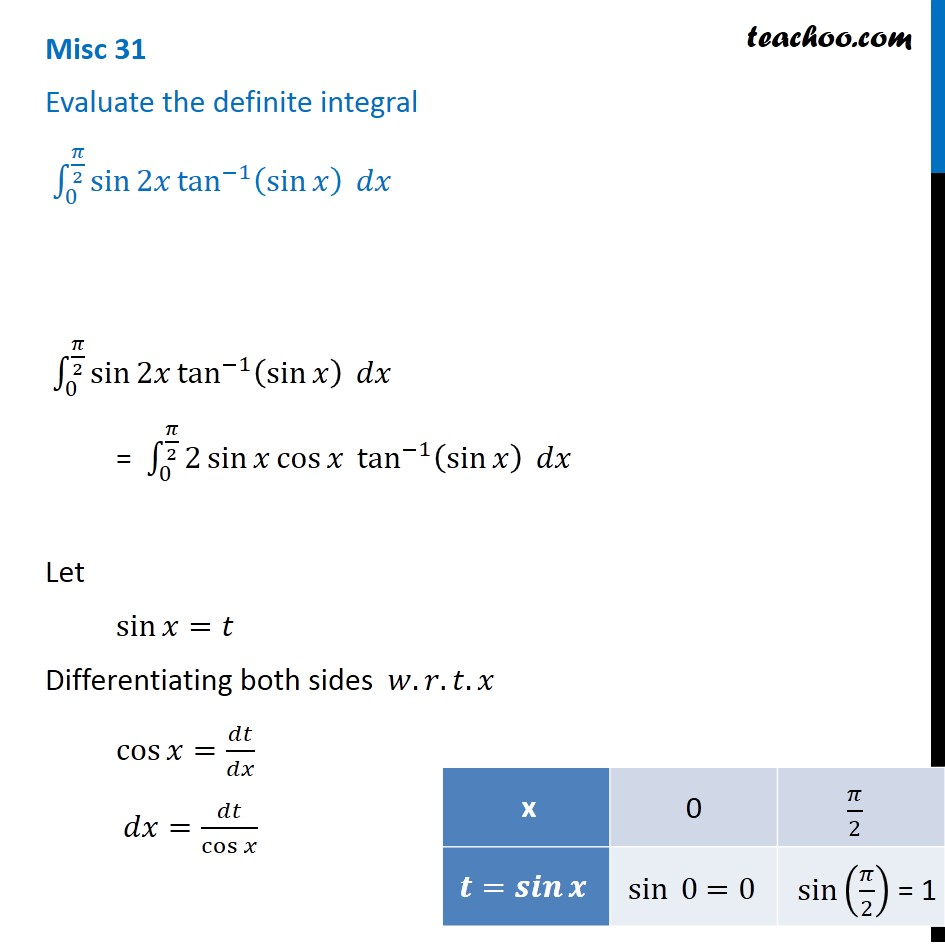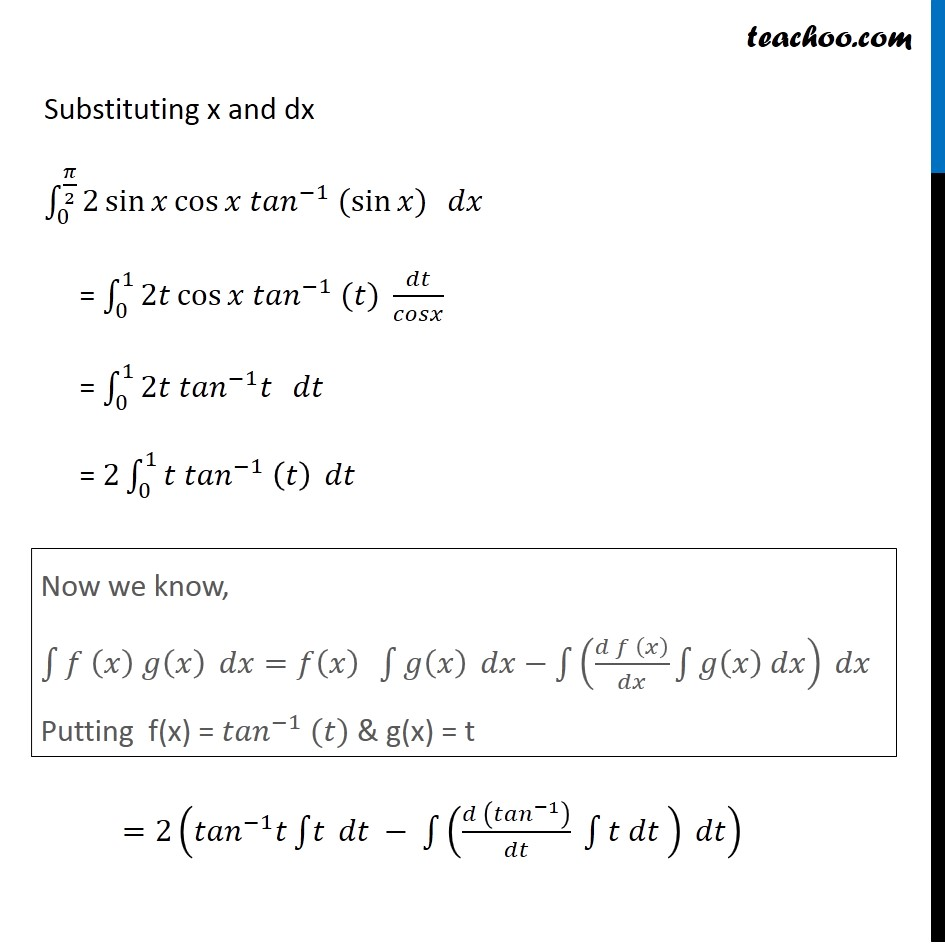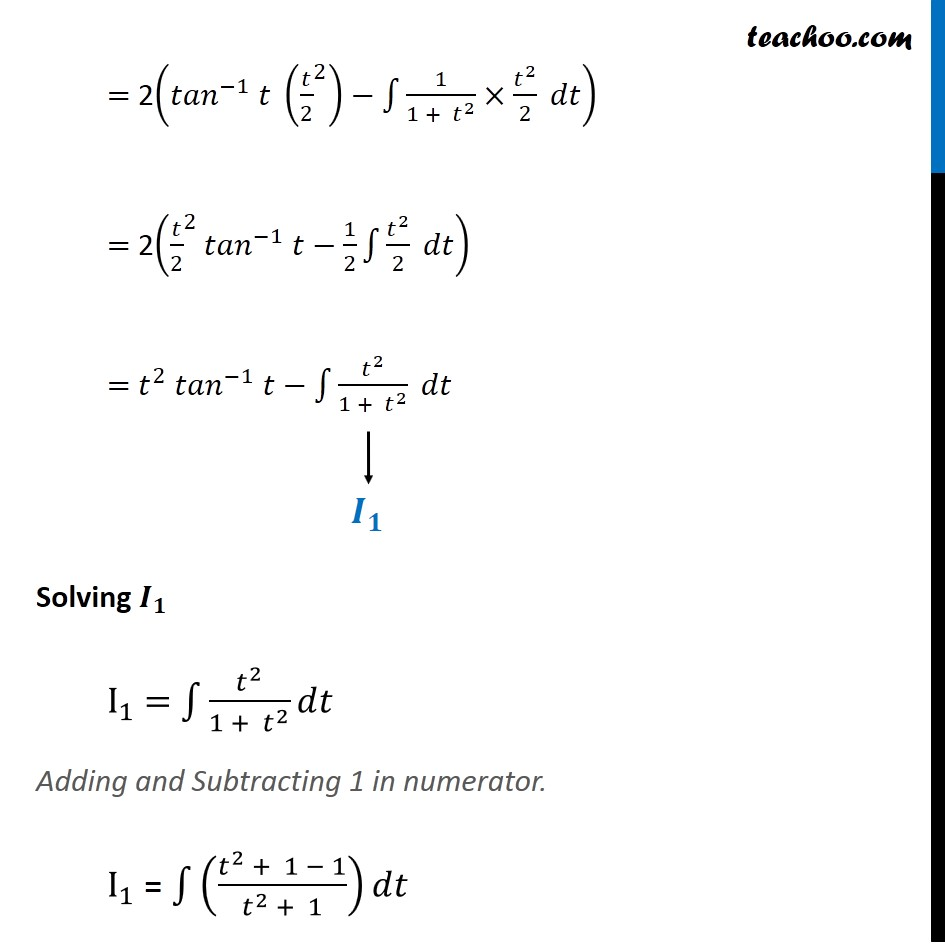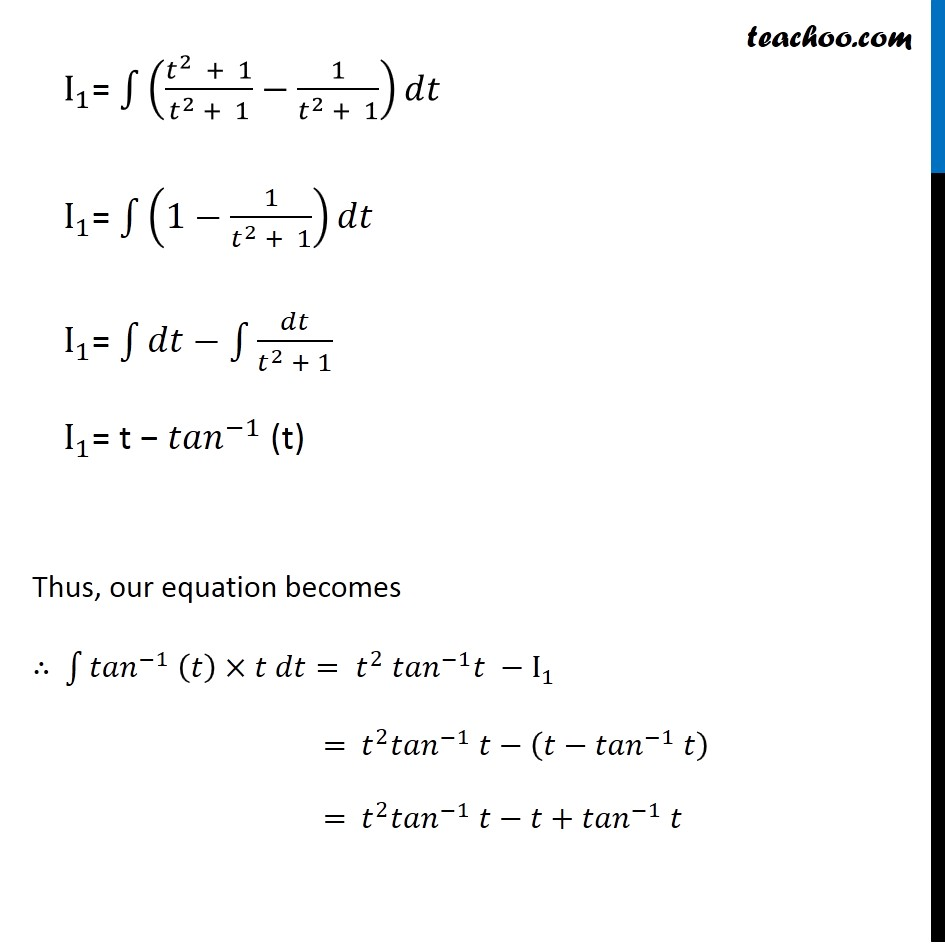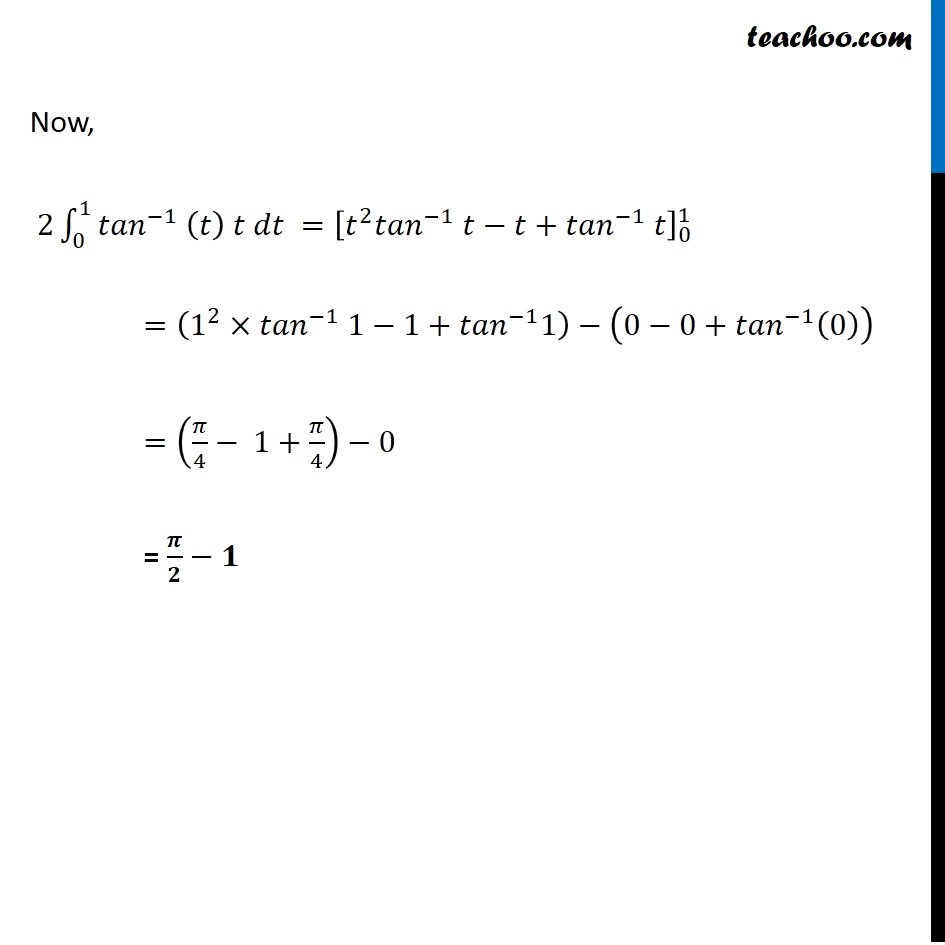Get live Maths 1-on-1 Classs - Class 6 to 12

### Transcript

Misc 31 Evaluate the definite integral ∫_0^(𝜋/2)▒〖sin⁡2𝑥 tan^(−1)⁡(sin⁡𝑥 ) 〗 𝑑𝑥 ∫_0^(𝜋/2)▒〖sin⁡2𝑥 tan^(−1)⁡(sin⁡𝑥 ) 〗 𝑑𝑥 = ∫_0^(𝜋/2)▒〖2 sin⁡𝑥 cos⁡𝑥 tan^(−1)⁡(sin⁡𝑥 ) 〗 𝑑𝑥 Let sin⁡𝑥=𝑡 Differentiating both sides 𝑤.𝑟.𝑡.𝑥 cos⁡𝑥=𝑑𝑡/𝑑𝑥 𝑑𝑥=𝑑𝑡/cos⁡𝑥 Substituting x and dx ∫1_0^(𝜋/2)▒〖2 sin⁡〖𝑥 cos⁡〖𝑥 〖𝑡𝑎𝑛〗^(−1) (sin⁡〖𝑥) 〗 〗 〗 〗 𝑑𝑥 = ∫1_0^1▒〖2𝑡 cos⁡〖𝑥 〖𝑡𝑎𝑛〗^(−1) (𝑡) 〗 〗 𝑑𝑡/𝑐𝑜𝑠𝑥 = ∫1_0^1▒〖2𝑡 〖𝑡𝑎𝑛〗^(−1) 𝑡 〗 𝑑𝑡 = 2∫1_0^1▒〖𝑡 〖𝑡𝑎𝑛〗^(−1) (𝑡) 〗 𝑑𝑡 =2(〖𝑡𝑎𝑛〗^(−1) 𝑡∫1▒𝑡 𝑑𝑡 − ∫1▒〖((𝑑 (〖𝑡𝑎𝑛〗^(−1) ))/𝑑𝑡 ∫1▒〖𝑡 𝑑𝑡 〗) 〗 𝑑𝑡) Now we know, ∫1▒〖𝑓 (𝑥) 𝑔(𝑥) 〗 𝑑𝑥=𝑓(𝑥) ∫1▒𝑔(𝑥) 𝑑𝑥−∫1▒〖((𝑑 𝑓 (𝑥))/𝑑𝑥 ∫1▒〖𝑔(𝑥) 𝑑𝑥〗) 〗 𝑑𝑥 Putting f(x) = 〖𝑡𝑎𝑛〗^(−1) (𝑡) & g(x) = t = 2(〖𝑡𝑎𝑛〗^(−1) 𝑡 (〖𝑡/2〗^2 )−∫1▒1/(1 + 𝑡^2 )×𝑡^2/2 𝑑𝑡) = 2(〖𝑡/2〗^2 〖𝑡𝑎𝑛〗^(−1) 𝑡−1/2 ∫1▒𝑡^2/2 𝑑𝑡) = 𝑡^2 〖𝑡𝑎𝑛〗^(−1) 𝑡−∫1▒𝑡^2/(1 + 𝑡^2 ) 𝑑𝑡 Solving 𝑰_𝟏 I_1 = ∫1▒𝑡^2/(1 + 𝑡^2 ) 𝑑𝑡 Adding and Subtracting 1 in numerator. I_1 = ∫1▒((𝑡^2 + 1 − 1)/(𝑡^2 + 1))𝑑𝑡 I_1= ∫1▒((𝑡^2 + 1)/(𝑡^2 + 1)−1/(𝑡^2 + 1))𝑑𝑡 I_1= ∫1▒(1−1/(𝑡^2 + 1)) 𝑑𝑡 I_1= ∫1▒〖𝑑𝑡−∫1▒𝑑𝑡/(𝑡^2 + 1)〗 I_1= t − 〖𝑡𝑎𝑛〗^(−1) (t) Thus, our equation becomes ∴ ∫1▒〖〖𝑡𝑎𝑛〗^(−1) (𝑡)×𝑡 𝑑𝑡〗= 𝑡^2 〖𝑡𝑎𝑛〗^(−1) 𝑡 −I_1 = 𝑡^2 〖𝑡𝑎𝑛〗^(−1) 𝑡−(𝑡−〖𝑡𝑎𝑛〗^(−1) 𝑡) = 𝑡^2 〖𝑡𝑎𝑛〗^(−1) 𝑡−𝑡+〖𝑡𝑎𝑛〗^(−1) 𝑡 Now, 2∫1_0^1▒〖〖𝑡𝑎𝑛〗^(−1) (𝑡) 𝑡 𝑑𝑡〗 =[𝑡^2 〖𝑡𝑎𝑛〗^(−1) 𝑡−𝑡+〖𝑡𝑎𝑛〗^(−1) 𝑡]_0^1 =(1^2×〖𝑡𝑎𝑛〗^(−1) 1−1+〖𝑡𝑎𝑛〗^(−1) 1)−(0−0+〖𝑡𝑎𝑛〗^(−1) (0)) =(𝜋/4− 1+𝜋/4)−0 = 𝝅/𝟐−𝟏